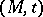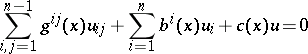# Huygens principle

Huygens' principle results from the mathematical fact that the solution of the wave equation at a pointof the three-dimensional space at the moment of timeis expressed in terms of the values of the solution and its derivatives on an arbitrary closed surface inside whichis contained, at preceding moments of time. In particular, the solution of the Cauchy problem at the pointfor the wave equation is determined only by the initial data at the intersection of the initial manifold with the characteristic cone ofand does not depend on the initial data inside and outside the characteristic cone (cf. also Characteristic surface). A rigorous mathematical formulation of Huygens' principle was first given by H. Helmholtz (1859) and by G. Kirchhoff (1882) for the stationary and non-stationary cases, respectively.(*)
for evendepends only on the initial data at the intersection of the initial manifold with the characteristic conoid if and only if the fundamental solution of (*) has no logarithmic terms, is a generalization of Huygens' principle to include the linear hyperbolic equation (*). For an account of the entire class of equations of the form (*) for which Huygens' principle is valid, see .